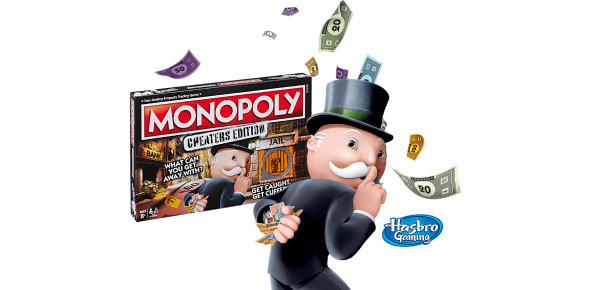# What Do You Know About Monopoly? Quiz

15 Questions | Total Attempts: 163SettingsMicro Economics CIA 3

• 1.
Monopolists are price takers
• A.

True

• B.

False

• 2.
A monopoly is the sole seller of a product with no close substitutes
• A.

True

• B.

False

• 3.
The demand curve facing a monopolist is the market demand curve for its product
• A.

True

• B.

False

• 4.
For the monopolist, marginal revenue is always less than the price of the good
• A.

True

• B.

False

• 5.
Price discrimination is only possible if there is no arbitrage
• A.

True

• B.

False

• 6.
Price discrimination can raise economic welfare because output increases beyond that which would result under monopoly pricing
• A.

True

• B.

False

• 7.
Universities are engaging in price discrimination when they charge different levels of tuition to poor and wealthy students
• A.

True

• B.

False

• 8.
Which of the following is not a barrier to entry in a monopolized market?
• A.

The government gives a single firm the exclusive right to produce some good

• B.

The cost of production make a single producer more efficient than a large number of producers

• C.

A key resource is owned by a single firm

• D.

A single firm is very large

• 9.
A monopolist maximizes profit by producing the quantity at which
• A.

Marginal revenue equals marginal cost

• B.

Marginal revenue equals price

• C.

Marginal cost equals price

• D.

Marginal cost equals demand

• E.

None of the above occurs

• 10.
The inefficiency associated with monopoly is due to
• A.

The monopoly's profits

• B.

The monopoly's losses

• C.

Overproduction of the good

• D.

Underproduction of the good

• 11.
Compared to a perfectly competitive market, a monopoly market will usually generate
• A.

Higher prices and higher output

• B.

Higher prices and lower output

• C.

Lower prices and lower output

• D.

Lower prices and higher output

• 12.
The monopolist's supply curve
• A.

Is the marginal-cost curve above average variable cost

• B.

Is the marginal-cost curve above average total cost

• C.

Is the upward-sloping portion of the average-total cost curve

• D.

Is the upward-sloping portion of the average variable cost

• E.

Does not exist

• 13.
Which of the following statements about price discrimination is not true?
• A.

Price discrimination can raise economic welfare

• B.

Price discrimination requires that the seller be able to separate buyers according to their willingness to pay

• C.

Perfect price discrimination generates a deadweight loss

• D.

Price discrimination increases a monopolist's profits

• E.

For a monopolist to engage in price discrimination, buyers must be unable to engage in arbitrage

• 14.
A monopoly is able to continue to generate economic profits in the long run because
• A.

Potential competitors sometimes don't notice the profits

• B.

There is some barrier to entry to that market

• C.

The monopolist is financially powerful

• D.

Antitrust laws eliminate competitors for a specified number of years

• E.

Of all of the above

• 15.
The supply curve for a monopolist is always positively sloped
• A.

True

• B.

False

Related TopicsBack to top